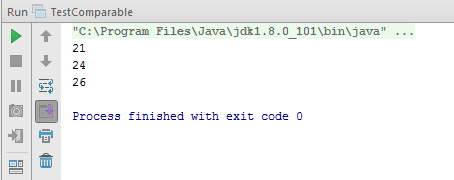# Learn about Comparable interface in Java

In Java, to check whether two objects are the same or not, we often use the equals() method to do this! To compare and sort two objects in an array or list, we can use the Comparable interface. In this tutorial, I will talk more about this interface for you to understand better!

OK, let’s get started.

Comparable interface is a generic interface that is used to sort objects that their class implements this interface.

For example, I have a Student object like this:

Now, I want to sort these Student objects in an array, the first thing I need to do is implement the Comparable interface for the Student object, like this:

When implementing this interface, we must define the method:

to compare this object with the object passed in the parameter of the method. The content of the method is ourself defined, but it is required to return the following values: negative, 0, and positive.

A negative number means that the object is smaller than the object passed in the method’s parameter, 0 means that the two objects are equal and the positive number means that the object is larger.

For the Student object in the above example, we can define the compareTo(Object other) method as follows:

OK, about the Comparable interface, you just have to grasp the above is enough! Now, to see how it works, I’m going to use the Student object above as an example.

In this example, we will use the TreeSet object to hold several Student objects. Because, the TreeSet object contains elements in sorted order, so when you add new Student objects, it will automatically rearrange them.

Code as follows:

Result: